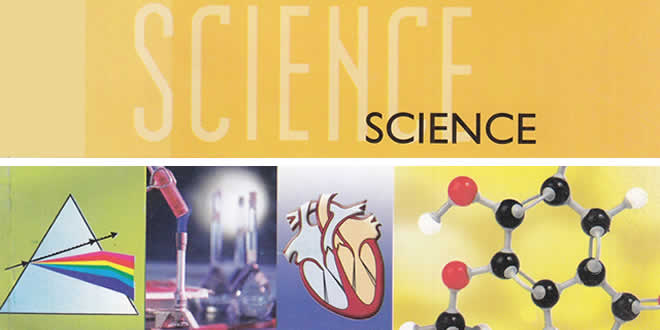Monday , August 8 2022# Metals and Non-metals MCQs: 10th Science Chapter 3

Metals and Non-metals MCQs: CBSE Class 10 Science Chapter 3 Metals and Non-Metals Multiple Choice Questions with Answers. MCQ Questions for Class 10 Science with Answers was Prepared Based on Latest Exam Pattern. Students can solve NCERT Class 10 Science Metals and Non-Metals Multiple Choice Questions with Answers to know their preparation level.

 Class: 10th Class Subject: Science Chapter: Chapter 03: Metals and Non-metals Quiz: 20 Questions

## Metals and Non-metals MCQs

### MCQs for Class 10 Science Metals and Non-metals with Answers & Explanation:

#### 1. Aluminium is used for making cooking utensils. Which of the following properties of aluminium are responsible for the same?

(i) Good thermal conductivity
(ii) Good electrical conductivity
(iii) Ductility
(iv) High melting point

(a) (i) and (ii)
(b) (i) and (iii)
(c) (ii) and (iii)
(d) (i) and (iv)

(a) Iron
(b) Aluminium
(c) Calcium
(d) Sodium

(b) Mercury
(c) Calcium
(d) Sodium

(a) Sonorousness
(b) Malleability
(c) Ductility
(d) Conductivity

(a) Al(OH)3
(b) Na2O
(c) NaAlO2
(d) AlNaO2

#### Zinc, Iron, Magnesium, Sodium

(a) Zinc > Iron > Magnesium > Sodium
(b) Sodium > Magnesium > Iron > Zinc
(c) Sodium > Zinc > Magnesium > Iron
(d) Sodium > Magnesium > Zinc > Iron

#### 7. Which of the following pairs will give dis-placement reactions?

(a) FeSO4 solution and Copper metal
(b) AgNO3 solution and Copper metal
(c) CuSO4 solution and Silver metal
(d) NaCl solution and Copper metal

#### 8. Non-metals form covalent chlorides because

(a) they can give electrons to chlorine
(b) they can share electrons with chlorine
(c) they can give electrons to chlorine atoms to form chloride ions
(d) they cannot share electrons with chlorine atoms

#### 9. Which of the following oxide(s) of iron would be obtained on prolonged reaction of iron with steam?

(a) FeO
(b) Fe2O3
(c) Fe3O4
(d) Fe2O3 and Fe2O4

Explanation: Reason: 3Fe (s) + 4H2O (g) → Fe3O4 (s) + 4H2 (g)

#### Metals and Non-metals MCQs – 10. Which of tire following are not ionic compounds?

(i) KCl
(ii) HCl
(iii) CCl4
(iv) NaCl

(a) (i) and (ii)
(b) (ii) and (iii)
(c) (iii) and (iv)
(d) (i) and (iii)

## Metals and Non-metals MCQs: 10th Science Chapter 3

(a) X2Y
(b) YZ
(c) XZ3</sub
(d) Y2Z

#### 12. The highly reactive metals like Sodium, Potassium, Magnesium, etc. are extracted by the

(a) electrolysis of their molten chloride
(b) electrolysis of their molten oxides
(c) reduction by aluminium
(d) reduction by carbon

(a) Sulphur
(b) Oxygen
(c) Nitrogen
(d) Iodine

(a) Na2O
(b) K2O
(C) Al2O3
(d) MgO

(a) Na2O
(b) CO
(c) CO2
(d) Al2O3

#### 16. The atomic number of an element ‘X’ is 12. Which inert gas is nearest to X?

(a) He
(b) Ar
(c) Ne
(d) Kr

Explanation: Reason: ‘X’ is Magnesium and Argon (Ar) with atomic number 12 is the closest inert gas to it.

(a) Roasting
(b) Reduction
(c) Calcination
(d) Smelting

#### 18. Oxides of moderately reactive metals like Zinc, Iron, Nickel, Tin, Copper etc. are reduced by using

(a) Aluminium as reducing agent
(b) Sodium as reducing agent
(c) Carbon as reducing agent
(d) Calcium as reducing agent

#### 19. In thermit welding a mixture of …… and …… is ignited with a burning magnesium ribbon which produces molten iron metal as large amount of heat is evolved.

(a) iron (III) oxide and aluminium powder
(b) iron (II) oxide and aluminium powder
(c) iron (III) chloride and aluminium powder
(d) iron (III) sulphate and aluminium powder

(a) Galium
(b) Aluminium
(c) Zinc
(d) Silver

## Metals and Non-metals MCQs: 10th Science Chapter 3

(a) Mg
(b) Na
(c) P
(d) Ca

#### Metals and Non-metals MCQs – 22. Reaction between X and Y forms compound Z. X loses electron and Y gains electron. Which of the following properties is not shown by Z?

(a) Has high melting point
(b) Has low melting point
(c) Conducts electricity in molten state
(d) Occurs as solid

Explanation: Reason: Z is an ionic compound. It has a high melting point.

#### 23. The electronic configurations of three elements X, Y and Z are X — 2, 8; Y — 2, 8, 7 and Z — 2, 8, 2. Which of the following is correct?

(a) X is a metal
(b) Y is a metal
(c) Z is a non-metal
(d) Y is a non-metal and Z is a metal

Explanation: Reason: According to the electronic configuration, Y is Chlorine and Z is Magnesium.

#### 24. Amalgam is an alloy of

(a) Copper and Tin
(b) Mercury
(d) Copper and Zinc

#### 25. Copper objects lose their shine and form green coating of

(a) Copper oxide
(b) Copper hydroxide and Copper oxide
(c) Basic Copper carbonate
(d) Copper carbonate